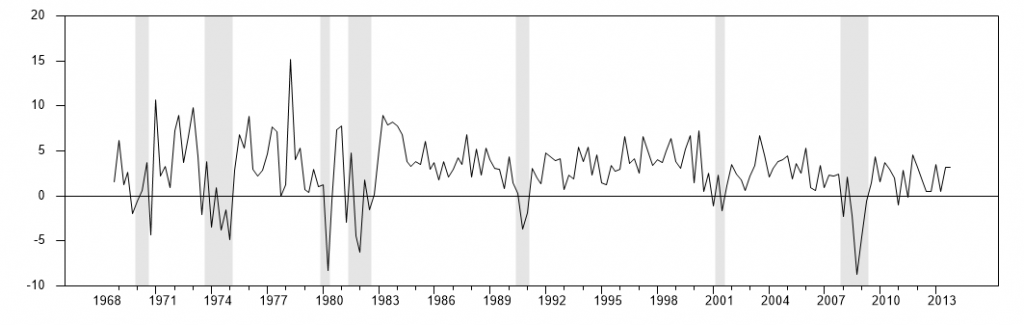Wednesday , September 23 2020Home / Econbrowser - James Hamilton / Guest Contribution: “A non-Gaussian macro world”

# Guest Contribution: “A non-Gaussian macro world”by
Menzie Chinn
My articles My siteMy booksAbout me
Summary:
Today, we are pleased to present a guest contribution written by Laurent Ferrara (Professor of International Economics, SKEMA Business School, Paris), and Director, International Institute of Forecasting. I recently discussed a very nice paper by Anna Orlik (FRB) and Laura Velkamp (NYU, Stern School of Business) on uncertainty shocks and the role of Black Swans, at the Paris School of Economics – SCOR Annual Conference. By playing with the data they used (real US GDP growth rate from 1968q4 to 2013q4, see Figure below), it is striking to see the degree of Non-Gaussianity of this series. Note: Quarterly changes in log real Gross Domestic Product for the US and NBER recessions, source Fred database (GDPC1). Indeed, there is an asymmetry to the left (Skewness= – 0.30) and the tails of the

Topics:
Menzie Chinn considers the following as important:

This could be interesting, too:

Menzie Chinn writes Implications of a “No Recovery Package” Outcome

Menzie Chinn writes Business Cycle Indicators, September 15th

Menzie Chinn writes September Economic Forecasts: Uncertainty Dominates at Year End

Menzie Chinn writes August Private Payrolls

Today, we are pleased to present a guest contribution written by Laurent Ferrara (Professor of International Economics, SKEMA Business School, Paris), and Director, International Institute of Forecasting.

I recently discussed a very nice paper by Anna Orlik (FRB) and Laura Velkamp (NYU, Stern School of Business) on uncertainty shocks and the role of Black Swans, at the Paris School of Economics – SCOR Annual Conference. By playing with the data they used (real US GDP growth rate from 1968q4 to 2013q4, see Figure below), it is striking to see the degree of Non-Gaussianity of this series.Note: Quarterly changes in log real Gross Domestic Product for the US and NBER recessions, source Fred database (GDPC1).

Indeed, there is an asymmetry to the left (Skewness= – 0.30) and the tails of the distribution are much thicker than those of a Gaussian distribution (Kurtosis = 4.98). A standard Jarque-Bera test strongly rejects the null of Gaussianity of this distribution.  When plotting the estimated empirical distribution of the GDP growth rate and a Normal distribution with corresponding estimated mean and variance, we clearly see the gap between the two distributions (see Figure below).Note: Kernel estimation of the US GDP growth using the R ‘density’ function with default options (black line). Sample mean is equal to 2.74 and sample standard error is equal to 3.30. Red line is the Gaussian distribution with those 2 estimated parameters.

In fact, the right tail of the empirical distribution seems to be quite in line with the one from a Gaussian distribution, but there are some clear divergences on the left tail, mainly due to the occurrence of seven recessions over the sample, as defined by the NBER Business Cycle Dating Committee.

By chance, econometricians have access to a battery of theoretical distributions accounting for asymmetry and fat tails. In a recent paper by Adrian, Boyarchenko and Giannone, just published in the AER, a Skewed-Student distribution is used to predict US GDP growth starting from a Financial Condition Index. This Skewed-Student distribution (see Azzalini and Capitanio, 2003) accounts for both asymmetry and fat tails using four parameters to be estimated, reflecting the first four moments of the distribution.

By fitting a Skewed-Student distribution to the US GDP growth through the Maximum Likelihood approach, we get indeed a much better fit than when using the Gaussian distribution (see Figure below)Note: Kernel estimation of the US GDP growth using the R ‘density’ function with default options. Sample mean is equal to 2.74 and sample standard error is equal to 3.30. Red line is the Gaussian distribution with those 2 parameters. Blue line is the fitted Skewed-Student distribution using the R package ‘sn’

Does it matter to account of non-Gaussianity? For example, assume we want to estimate the (unconditional) probability of a Black Swan. We define a Black Swan as in the paper by Orlik and Veldkamp: a Black Swan is negative event that occurs on average every 100 years. Using our data, this corresponds to a value of GDP growth equal to -6.53%, based on the Gaussian distribution (ie: a quarterly probability of 0.0025). However, if we think that the correct distribution is a Skewed-Student, the estimated unconditional probability of a Black Swan increases to 0.0130.  That is the unconditional probability of a Black Swan using a Skewed-Student distribution is more than 5 times higher than when using a Gaussian distribution.

A lesson from this small exercise at the current juncture is that recession probabilities estimated using Gaussian models are likely to be largely under-estimated.

This post written by Laurent Ferrara.He is Professor of Public Affairs and Economics at the University of Wisconsin, Madison